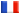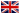# Séminaire de Cryptographie

## Cécile Pierrot### Simplified Settings for Discrete Logarithms in Small Characteristic Finite Fields

Public key cryptography is based on hard problems, such as the discrete logarithm problem (DLP). In this talk, I focus on the discrete logarithm problem in finite fields:

Given GF(q^k) and a generator g of GF(q^k)*, we say that we solve the DLP in GF(q^k) if, for any arbitrary element h in GF(q^k)*, we are able to recover an integer x such that: g^x = h.

When the characteristic is small compared to the extension degree, the best complexity that can be achieved is quasipolynomial in log(q^k). I present here a simplified version of this quasipolynomial algorithm that has several advantages:

1/ I swear it is simple, or at least I will do my best to make it understandable.

2/ Together with additional ideas, simplifying the original settings permits to decrease the complexity of relation collection, linear algebra and extension phases, that dominate in practice all discrete logarithms computations. Namely, the complexity is reduced from O(q^7) to O(q^6).

3/ With our simplified settings, the complexity achieved in the general case became similar to the complexity known for Kummer (or twisted Kummer) extensions. Thus it permitted to achieve a discrete log computation in GF_(3^(5*497)), that is not only the highest cardinality reached in characteristic 3, but also not a special extension field as previous target fields were.

This is a joint work with Antoine Joux.